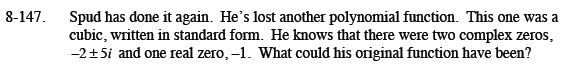### Home > CCA2 > Chapter 8 > Lesson 8.3.2 > Problem8-147

8-147.

Spud has done it again. He's lost another polynomial function. This one was a cubic, written in standard form. He knows that there were two complex zeros, −2 ± 5i and one real zero, −1. What could his original function have been? Homework Help ✎Use the three zeros to write the polynomial in factored form.

p(x) = (x − (− 1))(x − (−2 + 5i))(x − (−2 − 5i))

Multiply the two complex polynomials.

(x − (−2 + 5i))(x − (−2 − 5i))
x2 + 4x + 29

Multiply the result by (x + 1).

(x + 1)(x2 + 4x + 29)

p(x) = x3 + 5x2 + 33x + 29